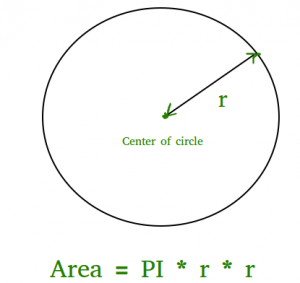GeeksforGeeks App
Open AppBrowser
Continue

# JavaScript program to find area of a circle

Given the radius of a circle, find the area of that circle. The area of a circle can simply be evaluated using the following formula:Area of circle

Where r is the radius of the circle and it may be in float because the value of the pie is 3.14

Approach: Using the given radius, find the area using the above formula: (pi * r * r) and print the result in float.

Example: Below is the example that will illustrate the program to find area of a circle:

## Javascript

 ``

Output:

`Area of Circle is: 78.53981633974483`

Time Complexity: O(1).
Auxiliary Space: O(1), since no extra space has been taken.

Another Approach: Using Math.Pi

We can get the value of pi using the Math module in javascript

Below is the Implementation:

## Javascript

 ``

Output:

`Area of Circle is: 78.53981633974483`

My Personal Notes arrow_drop_up